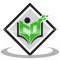## Stefan Boltzmann ConstantUpdated on 21-Dec-2022 15:47:01
Introduction The constant of Stefan Boltzmann is used to calculate the heat amount that is radiated by the blackbody. It preoccupied all the radiant energy that strikes it and releases the total radiated energy. In understanding the constant of Stefan Boltzmann's law, the blackbody is crucially important because that discusses the amount of power the sun provides off the provided temperature. This law constantly helps in analysing and examining the amount of heat and energy released by the earth into space. It permits scientists to examine and identify the amount of hotness of the sun that is based on the ... Read MoreUpdated on 13-Oct-2022 11:19:47
Introduction The radiation detector's development has been initiated with the transistor's invention. The recent detectors utilise calorimeters for the calculation of detected radiation energy. The plates of photography were utilised to recognise the left of tracks by the interactions of nuclei. The plate of photography can be placed in the way of a beam or material of radioactivity. Fogged may be visible or considered from the radiation exposure when the plate has been developed. In the earliest time, the electroscope was the main utilised radioactive detector. These were mainly used as a gold leaves pair that might get charged ... Read MoreUpdated on 13-Oct-2022 11:19:47
Introduction The gamma decay is a kind of radioactivity in which unsteady atomic nuclei gives out excess energy by a spontaneous electromagnetic process. The most notable form of gamma decay is gamma emission, gamma rays flows in the form of photons, and packets of electromagnetic energy of very short wavelength. Gama-decay also includes two key electromagnetic processes, internal pair production and internal conversion. In internal conversion excess energy present in the nucleus is directly transferred to one of its orbiting electrons and in the case of internal pair production excess amount of energy is converted in the particular electromagnetic field ... Read More

## Relation between Beta and Gamma FunctionUpdated on 13-Oct-2022 11:19:47
Introduction In physics, the relation of” beta and gamma functions” is popular to compute the various different functions. Gama is a type of single-variable function that represents the variable functions of the particles while beta is a type of dual variable function that is applicable for computing as well as representing the amplitude in terms of reggae trajectories. The relationship between the beta and gamma function is presented with the formula “B(p, q)=(Tp. Tq)/T(p+q)” In order to evaluate the integral functions of the gamma beta function, this formula is very crucial. What is the relation between beta and gamma function? ... Read MoreUpdated on 13-Oct-2022 11:19:47
Introduction The “radioactive beta decay” process exists in the field of nuclear physics. The radioactive process of decay is associated with the process of reaction in which beta ray is released from the “nucleus of an atom”. It helps in the transformation of the “original nuclides” to isobars” of the nuclide. In simple terms, in the process of “beta decay”, the “neutron” transforms into a “proton” through the “emission of an electron” which is further accompanied by “antineutrino”. The process of “beta decay” is resultant of a weak force which makes the “decay process” a relatively lengthy process. What is ... Read More